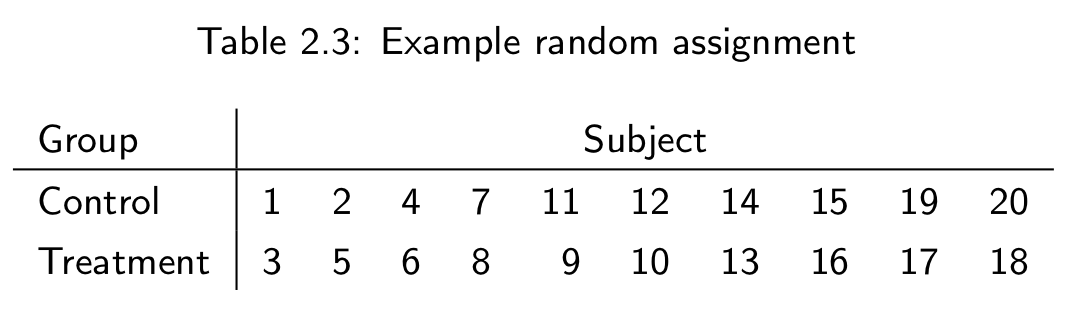This page gives a list of known issues in the 5.2 edition of A Portable Introduction to Data Analysis.

The errata are classified as being explanatory notes, simple typos or real mathematical errors.

If you find an error in the book then please email m.bulmer@uq.edu.au. The first person to report each error will receive a Freddo Frog. (If it is a mathematical error then you get a filled Freddo!).

 Page Type Description 22 Math Following the instructions on page 20, Table 2.3 should be92 Typo In Table 7.1, the basal oxytocin levels for Katie and Jana should be displayed as 4.40 and 4.50, respectively. 112 Typo "it is important to be able to reason about areas" 162 Math "The second number, 0.46, gives a height of 170.0 cm, and so on." 358 Typo The calculation for the second t statistic should be "(16.0 - 0)/(40.465/√15)", though the given value is correct.